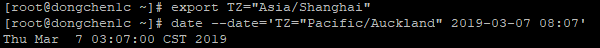Linux provides commands that allow you to view system’s time information, and with these commands, you can quickly get the time related information.

# System Time

We can use the date command to get or set the time of the system:

 ``````1 `````` ``date``

## Timezone

We can use the TZ variable to specify the time zone where our server is located:

 ``````1 2 `````` ``````export TZ=Pacific/Auckland date``````

## Time in Difference Timezone

Shows the time in Shanghai when a Auckland clock shows 8:07 on March 07, 2019:

 ``````1 2 `````` ``````export TZ="Asia/Shanghai" date --date='TZ="Pacific/Auckland" 2019-03-07 08:07'``````Changes timezone to China Shanghai and shows the corresponding time in Shanghai

## Hour - 24-hour format

 ``````1 `````` ``date +'%H'``

## Hour - 24-hour space padded format

 ``````1 `````` ``date +'%k'``

## Hour - 12-hour format

 ``````1 `````` ``date +'%I'``

## Hour - 12-hour space padded format

 ``````1 `````` ``date +'%l'``

## Minute

 ``````1 `````` ``date +'%M'``

## Second

 ``````1 `````` ``date +'%S'``

## Nanosecond

 ``````1 `````` ``date +'%N'``

## Seconds Since Epoch (Timestamp)

 ``````1 `````` ``date +'s'``

## Time - 24-hour format (Hour:Minute:Second)

 ``````1 `````` ``date +'%T'``

## Time - 24-hour format (Hour:Minute)

 ``````1 `````` ``date +'%R'``

## Locale Time - 24-hour Clock Time

 ``````1 `````` ``date +'%X'``

## Locale Time - 12-hour Clock Time

 ``````1 `````` ``date +'%r'``

## AM or PM - Uppercase

 ``````1 `````` ``date +'%p'``

## AM or PM - Lowercase

 ``````1 `````` ``date +'%P'``

## Timezone - Four Digits

 ``````1 `````` ``date +'%z'``

## Timezone - Nearest Minute

 ``````1 `````` ``date +'%:z'``

## Timezone - Nearest Second

 ``````1 `````` ``date +'%::z'``
 ``````1 `````` ``date +'%:::z'``
 ``````1 `````` ``date +'%Z'``